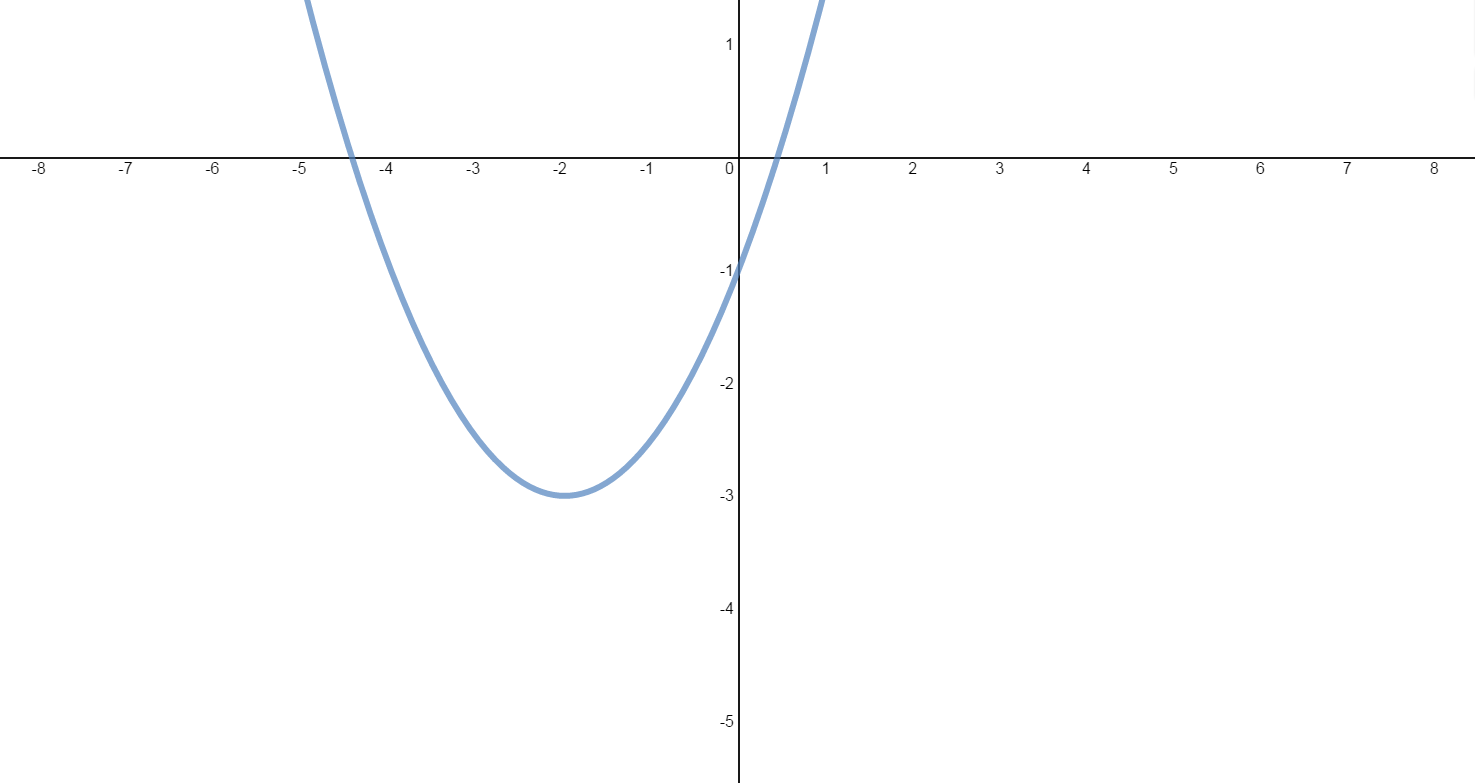# Slope and equation of tangent line

0/3
##### Introduction
###### Lessons
1. Connecting: Derivative & Slope & Equation of Tangent Line
Exercise: The graph of the quadratic function $f\left( x \right) = \frac{1}{2}{x^2} + 2x - 1$ is shown below.2. Find and interpret $f'\left( x \right)$.
3. Find the slope of the tangent line at:
i) $x = - 1$
ii) $x = 2$
iii) $x = - 7$
iv) $x = - 4$
v) $x = - 2$
4. Find an equation of the tangent line at:
i) $x = 2$
ii) $x = - 4$
iii) $x = - 2$
0/10
##### Examples
###### Lessons
1. Determining Equations of the Tangent Line and Normal Line
Consider the function: $f(x)=\frac{x}{32}(\sqrt{x}+{^3}\sqrt{x})$
1. Determine an equation of the tangent line to the curve at $x=64$.
2. Determine an equation of the normal line to the curve at $x=64$.
2. Locating Horizontal Tangent Lines
1. Find the points on the graph of $f(x)=2x^3-3x^2-12x+8$ where the tangent is horizontal.
2. Find the vertex of each quadratic function:
$f(x)=2x^2-12x+10$
$g(x)=-3x^2-60x-50$
3. Locating Tangent Lines Parallel to a Linear Function
Consider the Cubic function: $f(x)=x^3-3x^2+3x$
i) Find the points on the curve where the tangent lines are parallel to the line $12x-y-9=0$.
ii) Determine the equations of these tangent lines.
1. Determining Lines Passing Through a Point and Tangent to a Function
Consider the quadratic function: $f(x)=x^2-x-2$
1. Draw two lines through the point (3, -5) that are tangent to the parabola.
2. Find the points where these tangent lines intersect the parabola.
3. Determine the equations of both tangent lines.
2. Locating Lines Simultaneously Tangent to 2 Curves
$f(x)=x^2$
$g(x)=\frac{1}{4}x^2+3$
1. Sketch each parabola.
2. Determine the lines that are tangent to both curves.
###### Free to Join!
StudyPug is a learning help platform covering math and science from grade 4 all the way to second year university. Our video tutorials, unlimited practice problems, and step-by-step explanations provide you or your child with all the help you need to master concepts. On top of that, it's fun - with achievements, customizable avatars, and awards to keep you motivated.
• #### Easily See Your ProgressWe track the progress you've made on a topic so you know what you've done. From the course view you can easily see what topics have what and the progress you've made on them. Fill the rings to completely master that section or mouse over the icon to see more details.
• #### Make Use of Our Learning Aids###### Practice Accuracy

See how well your practice sessions are going over time.

Stay on track with our daily recommendations.

• #### Earn Achievements as You LearnMake the most of your time as you use StudyPug to help you achieve your goals. Earn fun little badges the more you watch, practice, and use our service.
• #### Create and Customize Your AvatarPlay with our fun little avatar builder to create and customize your own avatar on StudyPug. Choose your face, eye colour, hair colour and style, and background. Unlock more options the more you use StudyPug.
###### Topic Notes
The derivative of a function is interpreted as the slope of the tangent line to the curve of the function at a certain given point. In this section, we will explore the meaning of a derivative of a function, as well as learning how to find the slope-point form of the equation of a tangent line, as well as normal lines, to a curve at multiple given points.
Point-Slope Form of a line with slope m through a point $(x_1,y_1): m=\frac{y-y_1}{x-x_1}$

Tangent Line & Normal Line
The normal line to a curve at a particular point is the line through that point and perpendicular to the tangent line.